Senior author, Open Source enthusiast.
Sunday, September 4, 2016 at 08:51

# LibreOffice Writer Equation Editor: Writing Mathematical Formulas

If you are looking for equation editor for LibreOffice, then this article is for you. This introduces LibreOffice Math as Writer's equation editor to write mathematical expressions in a text document. We give some examples around some mostly used math formulas like fractions, limits, integrals, sigmas, and so on. We also add official book documentation (free) for further references. We hope this helps you a lot.

## Basic Ways

There are at least two ways to write mathematical formulas in LibreOffice Writer:

• Slow way: by using LibreOffice Math editor window (GUI).
• Fast way: by writing LibreOffice Math markup codes directly into Writer document.
This article covers both ways by mentioning examples. First part of this article explains the slow way (GUI) and second part explains the fast way (writing the codes directly).

## First Step

To write a formula in Writer, first, place your cursor and then open menu Insert > Object > Formula. By default, you will see new input window from LibreOffice Math appears below and on the left side you see math formula templates selection (Math's Elements). This is the first step you will always do every time you need to write a formula.

## Formula Templates

You will work frequently with this toolbox (called Elements) to choose formula from templates available:

Elements toolbox is categorized in 10 different groups:

1. Unary/Binary Operators: contains addition, multiplication, division, fractions, negation, and boolean formulas.
2. Relations: contains relational expressions such as is equal to, is greater to, is less than, toward, is congruent to, and so on.
3. Set Operations: contains numerical set-related expressions such as is in, is not in, owns, intersection, union, difference, subset, superset, and so on.
4. Functions: contains square root, exponential, ln, log, sin, cos, tan, arcsin, and so on.
5. Operators: contains limit, sigma, product, coproduct, integral, , and curve integral.
6. Attributes: contains accents such as double dot, line above, vector arrow, circumflex, acute, grave, text formattings, and colors.
7. Brackets: contains round, square, braces, angle, upper ceil, floow, single lines, double lines, braces top, braces bottom brackets.
8. Formats: contains superscript (left and right), subscript (left and right), superscript (top and bottom), new line, small gap, alignments, vertical stacks, and matrix.
9. Others: contains special symbols such as infinity, partially, nabla, dots, arrows, and so on.
10. Examples: contains simple and complex examples such as Einstiein's relativity formula and so on.

## 1. Writing Division

To write a simple division (fraction) you need to select Unary (Binary Operators) > select Division (Fraction) > go to bottom console > you see the {<?>} over {<?>} code that represents the math fraction formula > change exactly every <?> code into the number you want > click the text document. See the result.

Corresponding Tools:

Examples:

## 2. Writing Multiplication

Multiplication belongs to Unary/Binary Operators. There are three multiplication types: x, dot, and star. To add it select Unary/Binary Operators > select one type > edit the markup code > click the document > see the result.

Corresponding Tools:

Examples:

 Markup Code Result 1 1 cdot 2 2 1 times 2 3 1 * 2

## 3. Writing Exponent

To write exponent, visit Functions > select one, either power or exponential functions. Then edit the markup code. There is also a faster way to write an exponent expression, by using caret (`^`) character like n^{2}.

Corresponding Tools:

Examples:

 Markup Code Result 1 a^{b}2 2^{2} 3 x^{ y } 4 exp(1000)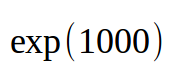## 4. Writing Square Root

To write a square root, choose between sqrt and nroot functions. They are belong to Functions category.

Corresponding Tools:

Examples:

 Markup Code Result 1 sqrt{144}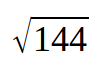2 nroot{3}{1000} 3 nroot{ y }{ x }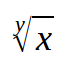4 nroot{ y }{ x + c }## 5. Writing Trigonometric Functions

To write sine, cosine, and tangent, visit Functions > select one function > edit the markup code.

Corresponding Tools:

Examples:

 sin(45)cos(45) tan(45)## 6. Writing Sigma

To write sigma, visit Operators > select sigma > edit the markup code.

Corresponding Tools:

Examples:

 Markup Code Result 1 sum from{m=1} to{4} n^{ 2 }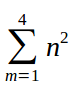2 sum from{n=1} to{10} ( 5k-4 ) = sum from{n=3} to{5} ( 10+7 ) + 943 sum from{x=1} to{n} k f( x ) = k sum from{x=1} to{n} f( x )## 7. Writing Limit

To write limit, visit Operators > select limit > edit the markup code.

Corresponding Tools:

Examples:

 Markup Code Result 1 lim from{x->a} f( x ) = L 2 lim from{x->0} { {3x} over {sin(4x)}}

## 8. Writing Integral

To write integral, visit Operators > select integral > edit the markup code. You may choose one from four types available. The only difference between them are their sub/superscript items.

Corresponding Tools:

Examples:

 int from{a} to{b} f( x ) dxint from{a} to{b} f( x )dx = F( b )-F( a )int sin(ax)  dx = -{{ 1 } over { a }} cos( ax ) +kint 2x^{3}dx = 2 over { 3+1 } x^{ 3+1 } +C = 1 over 2 x^{ 4 }+C## 9. Writing Logarithm

To write log, visit Functions > select log() > edit the markup code. To write a complete log expression (superscript added), visit Formats > select Superscript Left > edit the code.

Correspoding Tools:

Examples:

 Markup Code Result 1 log(7)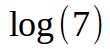2 {log(7)} lsup{10}3 { log(a times b) } lsup{p} = { log(a) } lsup{p} + { log(b) } lsup{ p }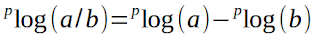4 { log ( a / b ) } lsup{p} = { log (a) } lsup{ p } - { log (b) } lsup{ p } newline newline## PART II

After you have been accustomed with LibreOffice Math interface, you will sense that this way of typing formulas is not fast. You will do open-close-open the window too many times. But once you have been accustomed with LibreOffice Math markup codes, you will work faster than using GUI.

For example there is a blank document in Writer. We want to write some mathematical questions for school students. It means we want to write mathematical text document.

To write such document, just write paragraph as usual, but write the Math markup codes directly to Writer > select the code > click the Formula button on the toolbar. See picture below.

Formula button is accessible via LibreOffice toolbar. If you can't see it, show it by doing right-click toolbar > Visible Buttons > click Formula. Clicking this button is the same as visiting menu Insert > Object > Formula